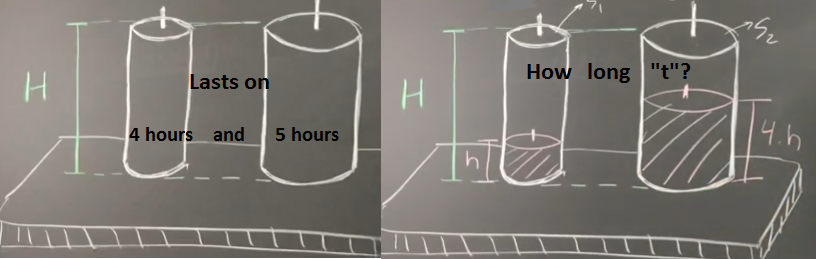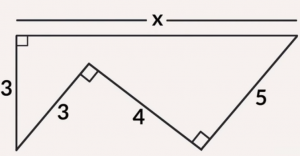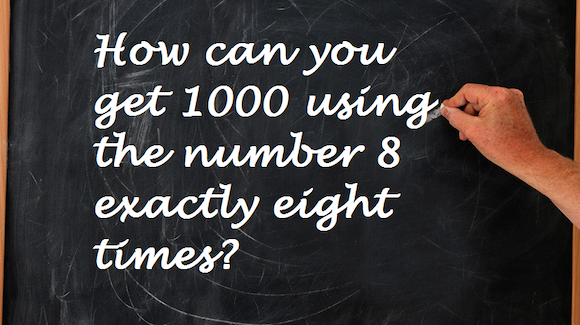# Math Challenges

Rules:

At the beginning of each month a Math challenge will come out.

To solve the challenge correctly, you have to:

• Write the solution with an explanation on a paper with your name and group and left it in the mailbox situated in the hall, before the first day of the following month.
• Write the reasoned solution in this website (at the bottom of the article).

There will be 3 categories:

• Pupils (ESO, Batxillerat and Professional Studies).
• Teachers and PAS.
• Web visitors.

## May challenge

This month we propose also two challenges :
1. The figure shows four identical rectangles located inside a square. The perimeter of each rectangle is 16 cm. What is the perimeter of the initial square?.
2. In a survey that was made of all the students in a school, 45 students said they were on foot, representing 60% of the responses. How many have said that they ride a bicycle, if we know that they are 12%?

May solutions:

• The perimeter of the starting square is 32 cm
• 9 people have used the bicycle

## April challenge

This month we propose two challenges :
1.    These days time passes differently and it seems that we live on another planet, we could call it Iris. One hour on planet Earth equals 3 days on Iris. Months on Iris last 5 weeks and have 6 days every week. How many Earth hours are 2 months on the Iris calendar?

1.   These days we have seen a women’s tennis tournament, the player who loses a match is eliminated. The results of the games that were played, are given in random order and are: Agnieska played with Simona, Garbiñe won over Venus, Serena won over Ana, Serena won over Garbiñe, Garbiñe won over Agnieska, Flavia won over Petra and Serena won over Flavia. Who played the tournament final?

`April solutions:`
`1. Two months on the planet Iris is 20h on Earth. `
`2. The final of the tournament will be between Serena and Garbiñe.`
Winners (pupils)
Noah B.
Winners (visitors)
Mar M.

## Mars challenge

Calculate the area of the shaded region, if ABCD is a square of 2 m side.
Solutions:
`If the side of the square measures 2, its area will be 4, so the area of the painted area would be half of the total, in this case 2.`

## February challenge

Calculate the time «t» that two candles have to be lit, of equal height but different diameter, so that one is 4 times the height of the other.
The thin one would be a total of 4 hours on and the thick 5 hours.Solutions:
The burn time is proportional to the volume of the candle, according to the ratio K = T / V (lit time / volume), k constant. If S is the surface of the base of each candle, it is fulfilled that 4/(S1 ·h) = 5 /(S2 ·h). You have to 4/5 · S2 = S1  (*) ( S is the surface of the base of each candle )

For the second situation to be fulfilled, t hours will have passed, then if one has to meet that one height  is 4 times the other: (4-t)/(S1·h) = (5-t)/(S2 · 4h) ; (*) (4 -t) / (⅘ · S2 ·h) = (5 -t) / S2 · 4h ; (4-t) / ⅘ = (5-t) / 4 ;

4 – t = ⅕ (5- t)  ; 20 – 5t = 5 – t ;  15 = 4t ; t = 3, 75 ; t = 3 h 45 ‘
Winners (pupils)
Ailén F (3rd eso), Mar B. (4th eso) and Pablo (1st Bac.)

## January challenge

Calculate X of the following figure. Be careful: don’t take anything for granted.Solutions:
Drawing parallels and using the Pythagorean Theorem twice is easily found x.
Winner (pupils)
4t d’ESO: Júlia P. B.
Winner (web visitors)
Alex Catalán

## December challenge

The first Math Challenge:Solutions:
Those are a couple of the many solutions:
• 888+88+8+8+8 = 1000
• [(8 x 8) + (8 x 8)] x 8 – (8 + 8 + 8) = 1000
Winners (pupils)
1st ESO: the whole 1.3 grup.
2nd  ESO: Isaac B. and  Marc R.,
3rd ESO: Jordi M., Eric V. and  Ailén F.
4th ESO:  Xavier G., Martina H., Olmo P. and  Alba V.
1st Bac.: Noah B., Marta A., Maria M. and  Chen R.
2nd Bac.: Paula S. and  Erin S.
Winners (teachers)
Núria B.
Winners (web visitors)
Ander Parra (also pupil) and Alex Catalán.